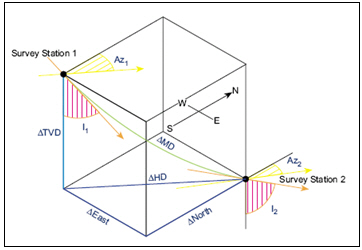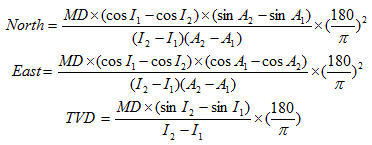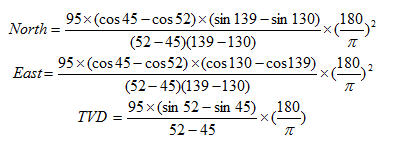Radius of Curvature Method is the most famous method for directional survey calculation. You can calculate North, East and TVD between directional surveys based on the Radius of Curvature Method as per formula below:Where;

MD = measured depth between surveys in ft

I1 = inclination (angle) at upper survey in degrees

I2 = inclination (angle) at lower in degrees

Az1= Azimuth direction at upper survey

Az2 = Azimuth direction at lower survey

Calculation example for Radius of Curvature Method

Survey 1

Depth = 7500 ft

Inclination = 45 degree (I1)

Azimuth = 130degree (A1)

Survey 2

Depth = 7595 ft

Inclination = 52 degree (I2)

Azimuth = 139 degree (A2)

MD = 7595 – 7500 = 95 ftAs per the calculation above,

North = -49.97 ft

East = 50.66 ft

TVD = 62.91 ft

Please find the Excel sheet used for directional survey calculation with Radius of Curvature Method.

Share the joyWorking in the oil field and loving to share knowledge.

### 6 Responses to Radius of Curvature Method

1.harris says:

You have a mistake in you TVD calculation equation for the radius of curvature method – you need to multiply nb 180/pi instead of (180/pi)^2

•DrillingFormulas.Com says:

Hi Harris,

Thanks very much for checking me. It is my mistake when I typed in up. However, the calculation sheet in this post is correct. I already double check it. I correct my post as well.

Cheers,
Rachain J.

2.puput says:

good morning, sir.

I have some trouble with my survey calculation in directional drilling. (Im a student)
Why i give some data is negative in vertical section from surface until KOP? what does the negative vertical section mean?

3.waqar Ahmed says:

this is very valuable data , could you please give me this kind of data in pdf so that i may easily download it to increase my DD knowledge . i’m a student

This site uses Akismet to reduce spam. Learn how your comment data is processed.